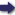(London :  Kegan Paul, Trench, Trübner & Co.,  1910.)

 Tools

## Search this bookPrev Page 59 Next``` CHAPTER LIV. 59 you get if you divide the second number by 60. How¬ ever, making this division, he got as quotient 73,051 and a remainder of f. Therefore he multiplied the whole by 4, in order that the fractions should be raised to wholes. For the same reason he also multiplies the following remainder by 4; but when he did not get wholes, as has been indicated, he returned to mul¬ tiplying by 60. Page 231. If we apply this method to a kalpa according to the theory of Brahmagupta, the first number, by which the remainder of the cycles is divided, is 131,493,037,500. The second number, by which the remainder of the signs of the ecliptic is divided, is 4,383,101,250. The third number, by which the remainder of the degrees is divided, is 73,051,687. In the remainder which we get by this division there is the fraction of ^. There¬ fore we take the double of the number, viz. 146,103,375, and we divide by it the double of the remainder of minutes. Brahmagupta, however, does not reckon by the kalpa Erahma- and caturyuga, on account of the enormous sums of piies this their days, but prefers to them the kaliyugct, in order tiiekaiiyuga to facilitate the calculation. Applying the preceding g'^rsnTIue'r method of aharga,na to the precise date of the kaliyugct, we multiply its sum of days by the star-cycles of a kalpa. To the product we add the bctsis, i.e. the remain¬ ing cycles which the planet had at the beginning of the kaliyuga. We divide the sum by the civil days of the kctliyuga, viz. 157,791,645. The quotient repre¬ sents the complete cycles of the planet, which are dis¬ regarded. The remainder we compute in the above-described manner, and thereby we find the mean place of the planet. The here-mentioned bctses are the following for the single planets:— ```Prev Page 59 Next